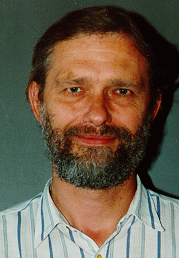24th Annual Geometry Festival in memory of Detlef Gromoll SUNY Stony Brook, Stony Brook, NY April 17-19, 2009Detlef Gromoll (1938-2008)
1. Jeff Cheeger, New York University
Quantitative Behavior of Maps from the Heisenberg Group to L1

Let (X,d) denote a metric space and let C denote a collection of metrics on X such that d'∈C implies c•d'∈C for any real number c>0. Put ρ(d,C)= infd'∈C inf{c: d' ≤ d ≤ c•d'}. Let L denote the collection of metrics on X of the form d'(x1,x2)=|f(x1)-f(x2)|L1, for some map f: X→L1, and let N denote the collection of metrics d on X such that (X,d1/2) embeds isometrically in L2. It is easy to verify that LN, and so ρ(d,N)≤ ρ(d,L). If X is finite with cardinality n, it was shown by Bourgain that ρ(d,L)=O(log n) for any metric d. Although the problem of computing ρ(d,L) exactly is equivalent to other fundamental problems for which there is believed to be no polynomial time algorithm, there is a quadratic time algorithm for computing ρ(d,N). Goemans and Linial conjectured that for some universal constant C>0, independent of n, ρ(d,N)≤ C • ρ(d,C). Their conjecture was refuted by Khot-Vishnoi (2005) who gave a sequence of examples for which the best C grows at least like a constant times log(log n). We will discuss a very difference sequence based on the Heisenberg group, for which C grows at least like (log n)^a for some explicit a. This is joint with with Kleiner and Naor. It is an outgrowth of ealier work of Lee-Naor and Cheeger-Kleiner.

2. Wolfgang Meyer, Westfälische Wilhelms-Universität Münster
The Contributions of Detlef Gromoll to Riemannian Geometry - Part 1

(presented by Blaine Lawson, Stony Brook)

The lecture will include D. Gromoll's contributions to the Differentable Sphere Theorem, the Diameter Rigidity Theorem, metric fibrations, the structure theory for complete manifolds of nonnegative sectional curvature, results for nonnegative Ricci curvature, and closed geodesics.

3. Wolfgang Meyer, Westfälische Wilhelms-Universität Münster
The Contributions of Detlef Gromoll to Riemannian Geometry - Part 2

(presented by Michael Anderson, Stony Brook)

The lecture will include D. Gromoll's contributions to the Differentable Sphere Theorem, the Diameter Rigidity Theorem, metric fibrations, the structure theory for complete manifolds of nonnegative sectional curvature, results for nonnegative Ricci curvature, and closed geodesics.

4. Marcos Dajczer, Instituto de Matematica Pura e Aplicada
Conformal Killing graphs with prescribed mean curvature

I will discuss the existence and uniqueness of graphs with prescribed mean curvature function over a bounded domain in Riemannian manifolds endowed with a conformal Killing vector field. The domain is contained in a hypersurface in the integrable orthogonal distribution and the graph is a hypersurface transversal to the flow lines of the field. This is joint work with Jorge H. Lira.

5. Guofang Wei, University of California Santa Barbara
Smooth Metric Measure Spaces

Smooth metric measure spaces are Riemannian manifolds with a conformal change of the Riemannian measure and occur naturally as measured Gromov-Hausdorff limit of Riemannian manifolds. The important curvature quantity here is the Bakry-Emery Ricci tensor, which corresponds to the (synthetic) Ricci curvature lower bound for (nonsmooth) metric measure spaces. What geometric and topological results for Ricci curvature can be extended to Bakry-Emery Ricci tensor? Recently there are many developments. We will discuss comparison geometry and rigidity in this direction.

6. Karsten Grove, University of Notre Dame
Positive Curvature: the Quest for Examples

We will discuss recent progress including the discovery and construction of new examples.

7. Christina Sormani, Lehman College and CUNY Graduate Center
The Intrinsic Flat Distance between Riemannian Manifolds

We define a new distance between oriented Riemannian manifolds that we call the intrinsic flat distance based upon Ambrosio-Kirchheim's theory of integral currents on metric spaces. Limits of sequence of manifolds, with a uniform upper bound on their volumes, the volumes of their boundaries and diameters are countably Hm rectifiable metric spaces with an orientation and multiplicity that we call integral current spaces. In general the Gromov-Hausdorff and intrinsic flat limits do not agree. However, we show that they do agree when the sequence of manifolds has nonnegative Ricci curvature and a uniform lower bound on volume and also when the sequence of manifolds has a uniform linear local geometric contractibility function. These results are proven using work of Greene-Petersen, Gromov, Cheeger-Colding and Perelman. We present an example of three manifolds with positive scalar curvature constructed using Gromov-Lawson connected sums attaching two standard 3 spheres with increasingly many tiny wormholes which converge in the Gromov Hausdorff sense to the standard three sphere but in the intrinsic flat sense to the 0 space due to the cancelling orientation of the two spheres. We conjecture this cannot occur if we exclude spaces with interior minimal surfaces. This is joint work with S. Wenger; transparencies and related preprints are available here

8. Gabriel Paternain, University of Cambridge
Transparent Connections over Negatively Curved Surfaces

Let M be a closed orientable surface of negative curvature. A unitary connection on a Hermitian vector bundle is said to be transparent if its parallel transport along closed geodesics of g is the identity. In this talk I will try to show that SU(2)-transparent connections can be understood in terms of Bäcklund transformations and that the trivial connection is locally unique.

9. Dedication of Detlef Gromoll Common Room